# Introduction to the pifpaf package: Estimating Potential Impact and Population Attributable Fractions from Cross-Sectional data

## Introduction

The Potential Impact Fraction (PIF) quantifies the contribution of risk-factor exposure to either morbidity (or mortality). In particular, it compares the observed burden of disease (or death) with a hypothetical counterfactual scenario. PIF is usually defined (1,2) for some exposure $$X\in \mathbb{R}^p$$ with parametrical Relative Risk $$RR(X;\theta)$$ with parameter $$\theta$$, and counterfactual function $$\textrm{cft}$$. If $$X$$ is categorical (discrete) then

$\begin{equation} \textrm{PIF} = \frac{\sum_{i=1}^m P_i \cdot RR(X_i;\theta) - \sum_{i=1}^m P_i \cdot RR\big(\textrm{cft}(X_i);\theta\big)}{\sum_{i=1}^m P_i \cdot RR(X_i;\theta)}, \end{equation}$

and if $$X$$ is continuous:

$\begin{equation} \textrm{PIF} = \frac{\int_{\mathbb{R}^p} RR(X;\theta)f(X)dX - \int_{\mathbb{R}^p} RR\big(\textrm{cft}(X);\theta \big)f(X)dX}{\int_{\mathbb{R}^p} RR(X;\theta)f(X)dX}. \end{equation}$

In the aforementioned equations $$P_i$$ represents the probability of $$X$$ being at the $$i$$-th category and $$f$$ the density function of $$X$$.

Some examples of Relative Risk functions include (3):

1. Linear: $$RR(X;\theta) = 1 + \theta X$$,
2. Exponential: $$RR(X;\theta) = e^{\theta X}$$,
3. Quadratic: $$RR(X;\theta_1, \theta_2) = 1 + \theta_1 X + \theta_2 X^2$$.

We remark that in both discrete and continuous cases, when the counterfactual is that of the “theoretical-minimum-risk-exposure” (i.e. the counterfactual corresponds to a Relative Risk of $$1$$) the PIF is equivalent to the Population Attributable Fraction (PAF) defined as:

$\begin{equation} \textrm{PAF} = \begin{cases} \frac{\sum_{i=1}^m P_i \cdot RR(X_i;\theta) - 1}{\sum_{i=1}^m P_i \cdot RR(X_i;\theta)} & \textrm{if } X \textrm{ is categorical}, \\ \\ \frac{\int_{\mathbb{R}^p} RR(X;\theta)f(X)dX - 1}{\int_{\mathbb{R}^p} RR(X;\theta)f(X)dX} & \textrm{if } X \textrm{ is continuous}. \\ \end{cases} \end{equation}$

In this document we present the pifpaf package which allows the estimation of both PIF and PAF when using information from cross-sectional data. This document is structured as follows:

1. We present a “quick start” guide if you really cannot resist the urge of using the package.
2. We briefly discuss the methods involved and their implementations.
3. We dive into deeper examples concerning more advanced options of our package so you are able to use it like a pro. These include estimation methods, confidence interval methods and plots.
4. We show some common error messages and how to fix them.

## Quick start

The basic ingredients for using the package are:

1. A Relative Risk function of the exposure $$RR(X;\theta)$$ which might depends on a parameter $$\theta$$.
2. A sample $$X_{(n)}$$ of $$n$$ exposure values (or if it is not available, at least estimated mean $$m$$ and variance $$s^2$$ of the exposure).
3. A consistent and asymptotically normal estimator $$\hat{\theta}$$ of $$\theta$$.
4. A function $$\textrm{cft}$$ that transforms the observed exposure into that of a counterfactual scenario.

If you have those ingredients you are ready to start with our examples which include: a complete sample of the exposure with continuous Relative Risks, a complete sample of the exposure with categorical Relative Risks, only mean and variance of exposure with continuous Relative Risks, and only mean and variance of exposure with categorical Relative Risks.

### Continuous RR example: ozone exposure

This example aims to estimate the PIF and PAF of ozone on children’s lung growth. The airquality dataset (included in R) has information on ozone levels (ppb) for New York City.

require(datasets)
ozone_exposure  <- na.omit(airquality$Ozone) ozone_exposure <- as.data.frame(ozone_exposure)  Furthermore, assume normalized sampling weights for ozone exposure are given by: sampling_weights <- c(rep(1/232, 58), rep(0.75/58, 58)) Suppose the Relative Risk of reduced lung growth given exposure is defined by: $\begin{equation} RR(X;\theta) = e^{\theta X/5}, \end{equation}$ where $$\theta$$ is estimated by $$\hat{\theta} = 0.17$$ with variance $$\sigma_\theta^2 = 0.00025$$: thetahat <- 0.17 thetavar <- 0.00025 We can code the Relative Risk function as: rr <- function(X, theta){ exp(theta*X/5) } Notice that the parameters should be $$X$$ and $$\theta$$ in that order. Never forget this! Now we are ready to estimate the Population Attributable Fraction: paf(X = ozone_exposure, thetahat = thetahat, rr = rr, weights = sampling_weights) ##  0.9169378 We can estimate the Potential Impact Fraction provided we have a counterfactual. Let’s assume we want to scale exposure to ozone in 50% and reduce it by $$1$$ ppb. The counterfactual function is: cft <- function(X){0.5*X - 1} Notice that the counterfactual is solely a function of the exposure $$X$$. We are now ready to compute the Potential Impact Fraction: pif(X = ozone_exposure, thetahat = thetahat, rr = rr, cft = cft, weights = sampling_weights) ##  0.7921392 No study is complete without confidence intervals. Let’s calculate the confidence intervals for both PAF and PIF: paf.confidence(X = ozone_exposure, thetahat = thetahat, thetavar = thetavar, rr = rr, weights = sampling_weights, nsim = 200) ## Lower_CI Point_Estimate Upper_CI ## 0.867368576 0.916937807 1.000000000 ## Estimated_Variance ## 0.001390907 pif.confidence(X = ozone_exposure, thetahat = thetahat, thetavar = thetavar, rr = rr, cft = cft, weights = sampling_weights, nsim = 200) ## Lower_CI Point_Estimate Upper_CI ## 0.6936673 0.7921392 0.9477064 ## Estimated_Variance ## 0.0047397 Several plots are available to enrich our study. We can plot the effect of the counterfactual: counterfactual.plot(X = ozone_exposure, cft = cft, weights = sampling_weights, n=250)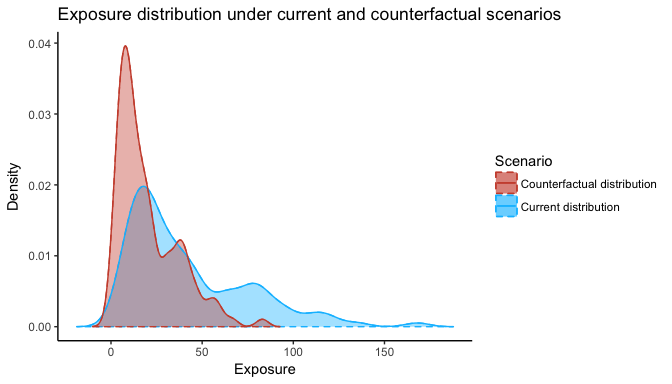We can also conduct several sensitivity analysis: 1. To see how the PAF changes as $$\theta$$ changes between $$0$$ and $$1/\pi$$ (notice that it is nonlinear). paf.plot(X = ozone_exposure, thetalow = 0, thetaup = 1/pi, rr = rr, weights = sampling_weights, mpoints = 25, nsim = 15)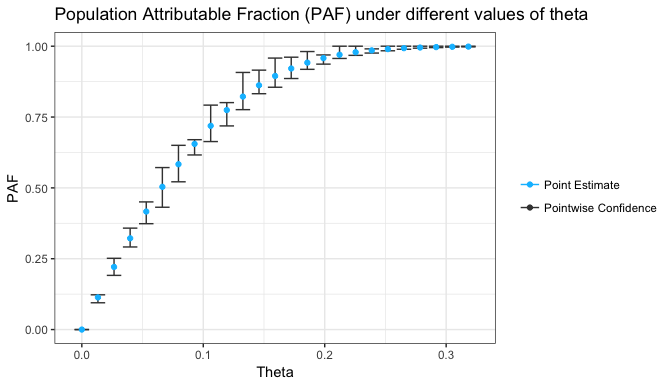The same plot is available for the PIF: pif.plot(X = ozone_exposure, thetalow = 0, thetaup = 1/pi, rr = rr, cft = cft, weights = sampling_weights, mpoints = 25, nsim = 15)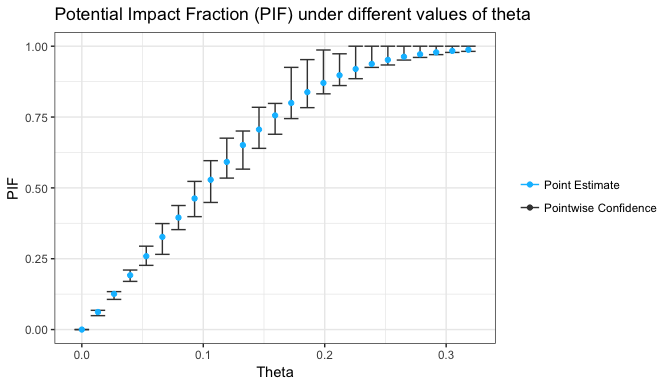1. To evaluate the robustness of the PAF (i.e. would it change much for a different sample?). paf.sensitivity(X = ozone_exposure, thetahat = thetahat, rr = rr, weights = sampling_weights, nsim = 10, mremove = 20)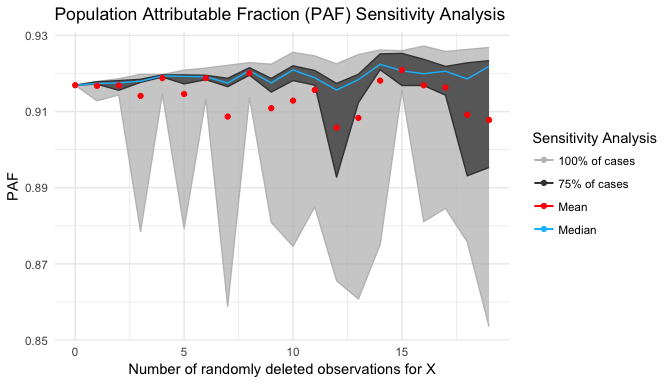The same can be done for the PIF: pif.sensitivity(X = ozone_exposure, thetahat = thetahat, rr = rr, weights = sampling_weights, nsim = 10, mremove = 20)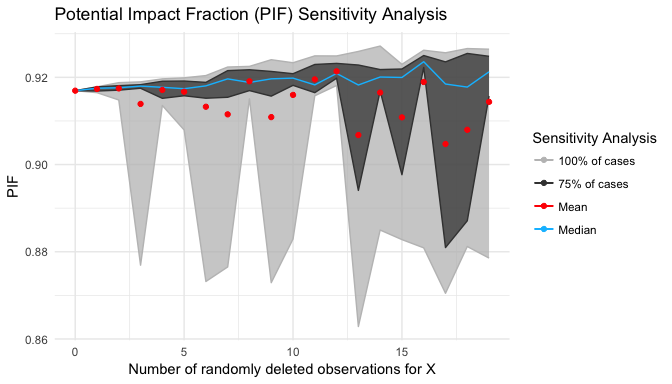1. To conduct a sensitivity analysis on how the PIF changes as the parameters of the counterfactual change. Notice that in order to use this function we need to specify in the definition of counterfactual the parameters involved (maximum 2): #Change the counterfactual function to specify the parameters involved cft_sensitivity <- function(X, a, b){a*X - b} We can also specify the range at which we will change the counterfactual’s parameters. For this example, let’s change $$b$$ from $$0$$ to $$1$$ and $$a$$ from $$0.5$$ to $$0.75$$. #Do the sensitivity analysis pif.heatmap(X = ozone_exposure, thetahat = thetahat, rr = rr, cft = cft_sensitivity, mina = 0.5, maxa = 0.75, minb = 0, maxb = 1, weights = sampling_weights, nmesh = 5)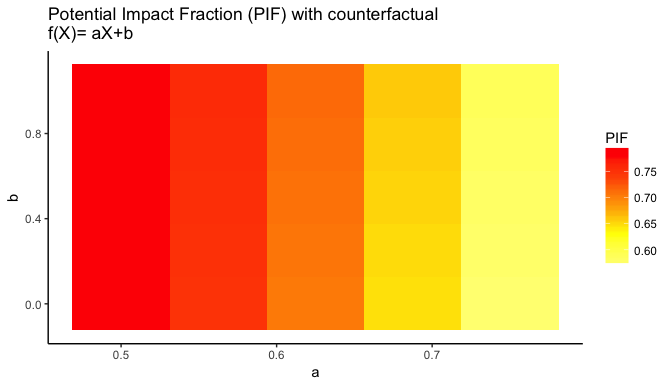### Discrete RR example: Tobacco consumption In this example we will compute the PIF and PAF of tobacco consumption over oesophageal cancer. For that purpose we will use the esoph dataset included in R. require(datasets) tobacco_consumption <- as.data.frame(esoph$tobgp)
This variable contains categorical information on the number of grams/day of tobacco consumed. Assume the Relative Risk function is given by: $\begin{equation} RR(X;\theta) = \begin{cases} \theta_1 & \textrm{ if consumption is } 0-9 \textrm{ g/day},\\ \theta_2 & \textrm{ if consumption is } 10-19, \\ \theta_3 & \textrm{ if consumption is } 20-29, \\ \theta_4 & \textrm{ if consumption is } 30_{+}. \\ \end{cases} \end{equation}$

with estimators $$\hat{\theta}_1 = 1$$, $$\hat{\theta}_2 = 1.59$$, $$\hat{\theta}_3 = 2.57$$, $$\hat{\theta}_4 = 4.11$$ of the respective $$\theta$$s. This can be programmed in R as follows:

#Thetas
thetahat <- c(1, 1.59, 2.57, 4.11)

#Relative Risk
rr       <- function(X, theta){

#Create empty vector to fill with RR's
r_risk <- rep(NA, nrow(X))

#Select by cases
r_risk[which(X == "0-9g/day")] <- theta
r_risk[which(X == "10-19")]    <- theta
r_risk[which(X == "20-29")]    <- theta
r_risk[which(X == "30+")]      <- theta

return(r_risk)
}

Notice that the Relative Risk assumes the exposure $$X$$ is a data.frame with each row representing an individual. We can estimate the Population Attributable Fraction:

paf(tobacco_consumption, thetahat, rr)
##  0.55047

Consider the counterfactual scenario where smokers in the categories $$20-29$$ and $$30_{+}$$ reduce their consumption to the $$10-19$$ category. This can be coded as:

cft <- function(X){

#Create empty matrix to fill with RR's
new_tobacco <- matrix(NA, nrow = nrow(X), ncol = 1)

#Select by cases
new_tobacco[which(X == "0-9g/day")] <- "0-9g/day"  #These remain
new_tobacco[which(X == "10-19")]    <- "10-19"     #the same
new_tobacco[which(X == "20-29")]    <- "10-19"
new_tobacco[which(X == "30+")]      <- "10-19"

# X in relative risk is received as a data.frame
new_tobacco <- as.data.frame(new_tobacco)
return(new_tobacco)
}

The Potential Impact Fraction is given by:

pif(tobacco_consumption, thetahat, rr, cft)
##  0.3575807
In order to compute confidence intervals, assume the following covariance matrix of $$\hat{\theta}$$: $\begin{equation} \Sigma_{\theta} = \left( \begin{array}{cccc} 0.119 & 0 & 0 & 0 \\ 0 & 0.041 & 0 & 0 \\ 0 & 0 & 0.001 & 0 \\ 0 & 0 & 0 & 0.093 \end{array} \right) \end{equation}$

which in R is:

thetavar <- diag(c(0.119, 0.041, 0.001, 0.093))

The confidence interval for the PAF is:

paf.confidence(X = tobacco_consumption, thetahat = thetahat, thetavar = thetavar, rr = rr,  confidence_method = "bootstrap", nsim = 200)
##           Lower_CI     Point_Estimate           Upper_CI
##        0.487164920        0.550469963        0.620128237
## Estimated_Variance
##        0.001339071

The confidence interval for the Potential Impact Fraction is given by:

pif.confidence(X = tobacco_consumption, thetahat = thetahat, thetavar = thetavar, rr = rr, cft = cft, confidence_method = "bootstrap", nsim = 200)
##           Lower_CI     Point_Estimate           Upper_CI
##        0.249247901        0.357580711        0.470490146
## Estimated_Variance
##        0.004015408

We remark that "bootstrap" is the only confidence_method designed for categorical relative risks.

The counterfactual.plot function produces an appropriate plot for the discrete exposure:

counterfactual.plot(tobacco_consumption, cft)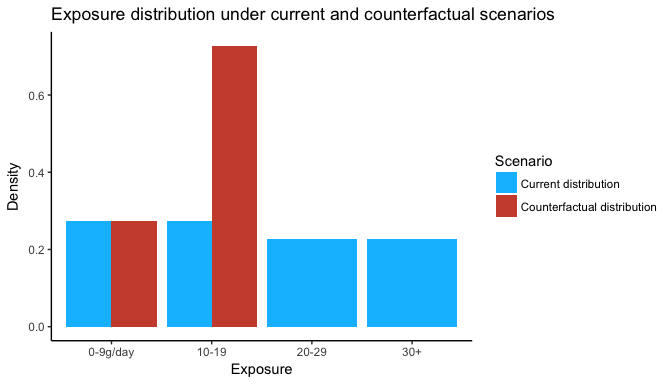A sensitivity analysis to evaluate both PAF and PIF’s robustness is available:

paf.sensitivity(tobacco_consumption, thetahat=thetahat, rr,
nsim = 10, mremove = 20)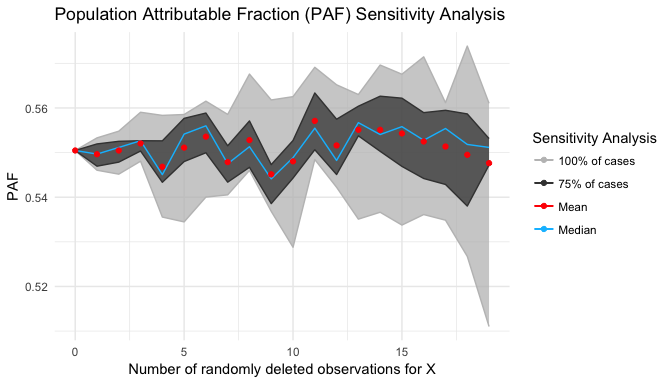pif.sensitivity(tobacco_consumption, thetahat=thetahat, rr=rr, cft = cft,
nsim = 10, mremove = 20)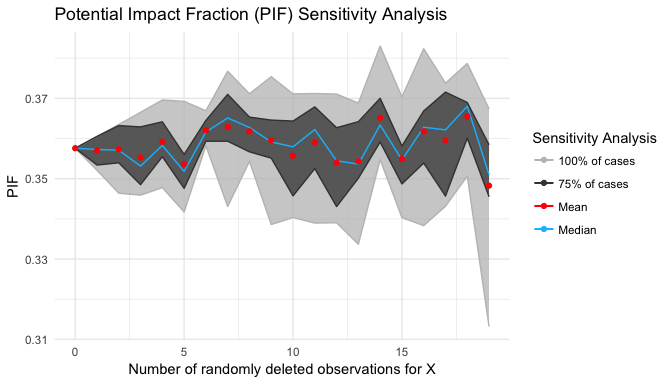### Incomplete data Continuous RR example: Systolic Blood Pressure

Consider the following data on Systolic Blood Pressure (SBP measured in mmHg) in females aged 30-44 by world region from (4):

sbp <- data.frame("Region"   = c("Afr D", "Afr E", "Amr A", "Amr B", "Amr D",
"Emr B", "Emr D", "Eur A", "Eur B", "Eur C",
"Sear B", "Sear D", "Wpr A", "Wpr B"),
"SBP_mean" = c(123, 121, 114, 115, 117, 126, 121,
122, 122, 125, 120, 117, 120, 115),
"SBP_sd"   = c(20, 13, 14, 15, 15, 15, 15,
15, 16, 17, 15, 14, 15, 16))
Furthermore, consider the Relative Risk of mortality given SBP as: $\begin{equation} RR(SBP; \theta) = 1 + \theta (SBP - 115)^2/121 \end{equation}$

with $$\hat{\theta} = 0.71$$ estimator of $$\theta$$ with estimated variance $$s^2 = 0.002$$. In R this is given by:

thetahat <- 0.71
thetavar <- 0.002

#Notice that the theoretical minimum risk value is 115 and not 0
rr       <- function(X, theta){ theta*(X - 115)^2/121 + 1} 

In this case, only mean and standard deviation information is available for each region. Terrible calamity! However the pifpaf package is prepared for such cases and the "approximate" method is in order. For example, let’s calculate the Population Attributable Fraction for the "Afr E" region:

#Get mean and variance
afr_mean <- as.data.frame(subset(sbp, Region == "Afr E")$SBP_mean) afr_var <- subset(sbp, Region == "Afr E")$SBP_sd^2

#Calculate paf using approximate method
paf(X = afr_mean, thetahat = thetahat, rr = rr, method = "approximate", Xvar = afr_var, check_rr = FALSE)
##  0.5460514

We can also compute confidence intervals:

paf.confidence(X = afr_mean, thetahat = thetahat,  thetavar = thetavar, rr = rr, method = "approximate", Xvar = afr_var, check_rr = FALSE, nsim = 200)
##                    Lower_CI              Point_Estimate
##                  -1.0019341                   0.5460514
##                    Upper_CI Estimated_Variance_log(PIF)
##                   0.8970649                   0.4268024

A counterfactual of reducing the overall SBP in 5 mmHg is given by:

cft <- function(X){X - 5}

The Potential Impact Fraction translates into:

pif(X = afr_mean, thetahat = thetahat, rr = rr, cft = cft, method = "approximate", Xvar = afr_var, check_rr = FALSE)
##  0.09322829

with confidence interval:

pif.confidence(X = afr_mean, thetahat = thetahat, rr = rr, cft = cft, method = "approximate", Xvar = afr_var, check_rr = FALSE, thetavar = thetavar, nsim = 200)
##                    Lower_CI              Point_Estimate
##                 -3.11298972                  0.09322829
##                    Upper_CI Estimated_Variance_log(PIF)
##                  0.80008826                  0.40486448

We can plot how PAF (and PIF) estimates change as functions of $$\theta$$:

paf.plot(X = afr_mean, thetalow = 0, thetaup = 1, rr = rr, method = "approximate", Xvar = afr_var,  check_rr = FALSE, mpoints = 25, nsim = 15)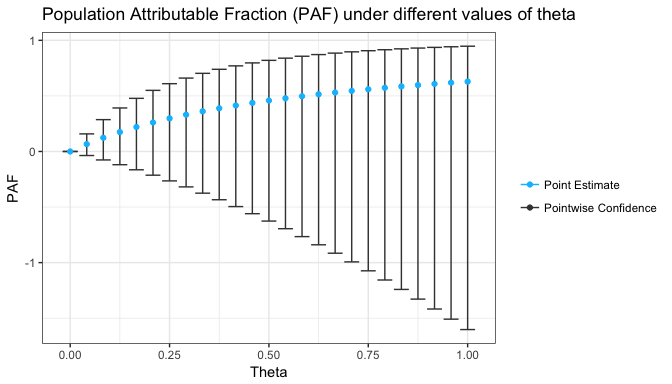pif.plot(X = afr_mean, thetalow = 0, thetaup = 1, rr = rr, cft = cft, method = "approximate", Xvar = afr_var,  check_rr = FALSE, mpoints = 25, nsim = 15)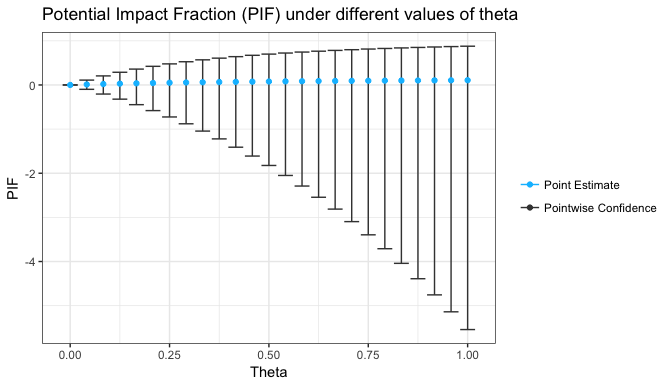### Incomplete data Categorical RR example: Body Mass Index

Consider the Relative Risk of dying associated to Body Mass Index (BMI) is given by: $\begin{equation} RR(BMI;\theta)=\begin{cases} 1 & \textrm{if } BMI<25,\\ 1.3\times BMI/25 & \textrm{if } 25\leq BMI<30,\\ e^{0.62\times BMI/30} & \textrm{if } BMI>30. \end{cases} \end{equation}$

Assume only the proportion of individuals in each category is known as well as the per-category mean and variance:

problem_data <- data.frame(Proportions   = c(  0.56,       0.21,       0.23),
Mean          = c(  23.2,       27.1,       31.9),
Variance      = c(  1.00,       0.87,       1.12))

rownames(problem_data) <- c("Normal", "Overweight", "Obese")

The approximate method as used in the previous example cannot be directly used as the Relative Risk function is non-differentiable (i.e. it is defined “by parts”). However we can compute the PAF for each category (Normal, Overweight and Obese) and then combine them. For that purpose, we define the Relative Risks for each category:

rr_normal     <- function(X, theta){theta}
rr_overweight <- function(X, theta){theta*X/25}
rr_obese      <- function(X, theta){exp(theta*X/30)}

and then compute the PAFs:

#Subpopulation PAF
paf_normal     <- paf(as.data.frame(problem_data["Normal","Mean"]), 1.00, rr = rr_normal, check_rr = FALSE, method = "approximate", Xvar = problem_data["Normal","Variance"])
paf_overweight <- paf(as.data.frame(problem_data["Overweight","Mean"]), 1.39, rr = rr_overweight, check_rr = FALSE, method = "approximate", Xvar = problem_data["Overweight","Variance"])
paf_obese      <- paf(as.data.frame(problem_data["Obese","Mean"]), 0.62, rr = rr_obese, check_rr = FALSE, method = "approximate", Xvar = problem_data["Obese","Variance"])

Finally the PAFs can be combined into the population PAF:

#Population PAF
paf.combine(c(paf_normal, paf_overweight, paf_obese), problem_data\$Proportions)
##  0.2431135

If pifs are estimated you can use the pif.combine function. Notice that in this case, no confidence intervals are available as no information on the correlation between the BMI categories is assumed.

## Methods

A broader definition of the Potential Impact Fraction (which includes both cases presented in the Introduction) is given by: $\begin{equation} \textrm{PIF} = 1 - \frac{E_{X}\left[RR\big(\textrm{cft}(X);\theta\big)\right] }{E_{X}\left[RR\big(X; \theta\big)\right]}, \end{equation}$

where $$\textrm{cft}(X)$$ denotes the counterfactual transform of the exposure $$X$$, $$RR$$ the relative risk function with parameter $$\theta$$. Note that the PAF is a special case of the $$\textrm{PIF}$$ when the counterfactual scenario corresponds to the one of the theoretical minimum risk exposure ($$RR=1$$). We have developed three methods of estimation: empirical, kernel and approximate.

### The Empirical Potential Impact Fraction

Assume a Relative Risk $$RR:\mathcal{X} \times \Theta \to I \subseteq (0,\infty)$$ for exposure $$X$$ and with parameter $$\theta$$. Let $$X_1, X_2, \dots, X_n$$ be a random sample of exposure and covariates $$X\in\mathcal{X}\subset\mathbb{R}^p$$ with normalized sampling weights $$w_1, w_2, \dots, w_n$$ and $$\hat{\theta} \in \Theta \subseteq \mathbb{R}^q$$ estimator of $$\theta$$ with $$\Theta, \mathcal{X}$$ compact sets. Define the functions:

$\begin{equation} \hat{\mu}_n^{\textrm{obs}}(\theta) = \sum\limits_{i=1}^{n} w_i RR\big( X_i; \theta \big), \quad \textrm{and} \quad \hat{\mu}_n^{\textrm{cft}}(\theta) = \sum\limits_{i=1}^{n} w_i RR\big( \textrm{cft}(X_i); \theta \big), \end{equation}$

then:

$\begin{equation}\label{pafestimate} \widehat{\textrm{PIF}} = 1 - \dfrac{\hat{\mu}_n^{\textrm{cft}}(\hat{\theta})}{\hat{\mu}_n^{\textrm{obs}}(\hat{\theta})}, \qquad \textrm{and} \qquad \widehat{\textrm{PAF}} = 1 - \dfrac{1}{\hat{\mu}_n^{\textrm{obs}}(\hat{\theta})} \end{equation}$

are Fisher-consistent estimators of the PIF and the PAF if $$\hat{\theta}$$ is Fisher-consistent. Furthermore if the Relative Risk $$RR$$ is either convex, concave or Lipschitz continuous as a function of $$\theta$$ and $$\hat{\theta}$$ is (asymptotically) consistent the estimators have asymptotic consistency.

### Kernel Based Potential Impact Fraction

Define the Relative Risk $$RR:\mathcal{X} \times \Theta \to I \subset (0,\infty)$$ (the additional hypotheses used for the empirical method are not necessary). Let $$\hat{f}$$ denote a kernel density obtained from the random sample of $$X\in\mathcal{X}\subseteq\mathbb{R}^p$$. Let $$\hat{\theta} \in \Theta \subset \mathbb{R}^q$$ be a consistent estimator of $$\theta$$. We define the functions:

$\begin{equation} \hat{\nu}_n^{\textrm{obs}}(\theta) = \int\limits_{\mathbb{R}^p} RR( x; \theta)\hat{f}(x)dx, \quad \textrm{and} \quad \hat{\nu}_n^{\textrm{cft}}(\theta) = \int\limits_{\mathbb{R}^p} RR\big( \textrm{cft}(x); \theta\big)\hat{f}(x)dx, \end{equation}$

then:

$\begin{equation} \widehat{\textrm{PIF}} = 1 - \frac{\hat{\nu}_n^{\textrm{cft}}(\hat{\theta})}{\hat{\nu}_n^{\textrm{obs}}(\hat{\theta})} \end{equation}$

is a consistent estimator of the Potential Impact Fraction ($$\textrm{PIF}$$).

### Approximate Empirical Potential Impact Fraction

Sometimes researchers do not have a random sample of the exposure $$X$$; nevertheless, they possess $$m$$, $$s^2$$ estimators of the exposure’s mean and variance (respectively). Furthermore, assume that for each $$\theta \in \Theta$$ the Relative Risk function $$RR(\cdot, \theta)$$ has a second order Taylor Expansion for all $$X \in \mathcal{X}$$ and that the counterfactual function is twice differentiable. An approximate point estimate for the PIF is given by the Laplace approximation:

$\begin{equation} \widehat{\textrm{PIF}}= 1-\frac{RR\big(\textrm{cft}(m),\theta\big) + \frac{1}{2}\sum_{i=1}^n\sum_{j=1}^n \textrm{Cov}(X_i,X_j)\frac{\partial^2 RR\big(\textrm{cft}(X),\theta\big)}{\partial X_i \partial X_j}\Big|_m}{RR(m;\hat{\theta})+\frac{1}{2} \sum_{i=1}^n\sum_{j=1}^n \textrm{Cov}(X_i,X_j)\frac{\partial^2 RR\big(X,\theta\big)}{\partial X_i \partial X_j}\Big|_m}. \end{equation}$

The approximate method solely requires the sample mean $$m$$ and variance $$s^2$$, not the whole sample. If the sample is available, the other methods should be preferred.

### Methods in code

All methods have been coded in the method option of the functions paf, pif and related. That is: we can estimate the PIF by different methods specifying the type:

#Data
set.seed(2374)
X        <- as.data.frame(rlnorm(100))
rr       <- function(X, theta){theta*X + 1}
cft      <- function(X){sqrt(X + 1)}
thetahat <- 0.1943

#Empirical
pif(X, thetahat, rr, cft, method = "empirical")
##  0.01022383
#Kernel
pif(X, thetahat, rr, cft, method = "kernel")
##  0.01054417
#Approximate
meanX <- as.data.frame(mean(X[,1]))
pif(meanX, thetahat, rr, cft, method = "approximate", Xvar = var(X))
##  0.01499538

Note that for the approximate method the correct input is mean and variance of X. If no method is specified in pif(X, thetahat, rr, cft) the "empirical" is chosen.

### Confidence Intervals

The "bootstrap" confidence method is the recommended method to calculate confidence intervals while using the kernel and empirical point estimate. However this method cannot be used for the approximate point estimate, since only mean and variance are available. Therefore other methods such as the "linear"´ and“loglinear”were developed to calculate the confidence interval of the PIF. The“inverse”and“one2one” methods can be used for some cases of the PAF resulting in additional precision. All, but the one to one method, consider $$\hat{\theta}$$ to be a consistent estimator of $$\theta$$ such that it is asymptotically normal with mean $$\theta$$ and variance $$\sigma_{\theta}^2$$ where $$\hat{\sigma}_{\theta}^2$$ is an estimator of $$\sigma_{\theta}^2$$. The following table shows the methods to estimate confidence intervals and when each of the methods can be used.

Confidence Interval Point Estimate PAF or PIF Extra Assumptions
Bootstrap Empirical & Kernel PIF & PAF None
Linear Empirical & Appproximate PIF & PAF None
Loglinear Empirical & Appproximate PIF & PAF None
Inverse Empirical & Appproximate PAF None
One to one Empirical & Appproximate PAF $$E_X\big[RR(X,\theta)\big]$$ is injective in $$\theta$$

Remember that to calculate the point estimate in the case of the approximate method the relative risk function $$RR(X,\theta)$$ and the counterfactual function $$\textrm{cft}(X)$$ must be continuously differentiable in terms of $$X$$. To get the confidence intervals of the PIF we calculate the variance of PIF (or of a transformation $$f(\textrm{PIF})$$). Notice that uncertainty comes from two sources: the exposure $$X$$ and the Relative Risk’s parameter $$\theta$$. The estimation process is done in three steps for the methods: linear, loglinear, and inverse:

1. We estimate the variance and expected value of $$f(\textrm{PIF})$$ as a function of $$\theta$$.
2. We use the fact that $$\theta$$ is asymptotically normal to estimate the unconditional variance:
$\begin{equation}\label{conditioningvar} \textrm{Var} \left(A \right) = E_{B} \left[ \textrm{Var} \left( A \left. \right| B \right) \right] + \textrm{Var}_{B} \left[ E\left( A \left. \right| B\right) \right]. \end{equation}$
1. We assume asymptotic normality of $$f(\widehat{\textrm{PIF}})$$ to estimate confidence intervals.

Further explanation of each of the methods is given below.

#### Bootstrap

Bootstrap consists on resampling with replacement several times from a given random sample. In this case from the random sample of exposure values $$X_1,X_2,\cdots X_n$$. For each re-sample $$X^{j}=X_1^{j}, X_2^j, \cdots, X_n^{j}$$ a value $$\theta_j$$ is simulated from a normal distribution with mean $$\hat{\theta}$$ and variance $$\hat{\sigma}^2_{\theta}$$. For each $$X^j$$ and $$\theta_j$$, $$\widehat{\textrm{PIF}}_j$$ is estimated with the selected method (empirical or kernel). From the $$\widehat{\textrm{PIF}}_j$$s a confidence interval for $$\textrm{PIF}$$ is calculated using the pivotal method (5).

#### Linear

The linear method considers Taylor’s first order approximation (linearization) of $$\widehat{\textrm{PIF}}$$ and the variance for the $$\widehat{\textrm{PIF}}$$ is calculated as the variance of the linearization. This approach is better known as the Delta Method (6).

#### Loglinear

The loglinear method uses the $$(1-\alpha)\times 100\%$$ confidence interval for $$\textrm{log}(1-\textrm{PIF})$$. The transformation $$1-e^{y}$$ (a one to one function) ensures that the confidence interval is at least $$(1-\alpha)\times 100\%$$ (7).

#### Inverse

The inverse method can be used only for confidence intervals of the $$\textrm{PAF}$$. The confidence interval ($$\textrm{IC}_{RR}$$) for $$E[RR(X,\theta)]$$ is calculated and then transformed to a confidence interval of $$\textrm{PAF}$$ by $$1-1/\textrm{IC}_{RR}$$. Once again (as in the loglinear case) the transformation $$1-1/x$$ is injective and thus the transform confidence interval of $$1 - 1/\textrm{IC}_{RR}$$ is at least $$(1-\alpha)$$ for the $$\textrm{PAF}$$ (7).

#### One to one

The one to one method is similar to the inverse method, since the confidence interval $$\textrm{IC}_{RR}$$ is calculated. The difference lies on how uncertainty on $$\theta$$ is calculated. The inverse method uses simulations of $$\theta$$, while the one to one method considers the upper and lower bounds of a $$1-\beta$$ confidence interval of $$\theta$$ to calculate a $$1-\alpha$$ confidence interval $$\textrm{IC}_{RR}$$, where $$\alpha>\beta$$. This method can only be used if $$E[RR(X;\theta)]$$ is injective in $$\theta$$ (7).

#### Extra option: force.min

For the inverse and one to one confidence intervals the option force.min is available. This option guarantees that the lower bound of the confidence intervals of the expected relative risk takes values greater or equal to one. This option is not recommended, as in most cases there is uncertainty on whether the relative risk can be less than 1 (albeit with “small” probability). However this option can be useful when one is absolutely certain the relative risk can’t be less than one.

This section is concerned with more advanced options of the pifpaf package functions. We first analyze how to choose an estimation method; secondly, we show how to choose a confidence interval; finally we show how to work with the plots.

### Deciding on the estimation method

The previous section discussed the three estimation methods used in the package. In this section we discuss some advanced options as well as how to choose the method.

#### Method choosing

Method Exposure Relative Risk $$\theta$$ Estimator
Kernel Continuous Continuous (One dimensional in pifpaf package) Asymptotically consistent
Empirical Continuous or Discrete Convex, Concave, or Lipschitz Asymptotically consistent
Empirical Continuous or Discrete Any Fisher consistent
Approximate Only mean and variance Twice differentiable & Convex, Concave, or Lipschitz Asymptotically consistent
Approximate Only mean and variance Twice differentiable Fisher consistent

#### Kernel

A kernel density is an approximation to the probability density function of a random variable constructed from the variable’s sample. For instance the following image shows the real density for a normally distributed random variable with mean $$0$$ and variance $$1$$ as well as the kernel approximation to said density from a sample of size 45.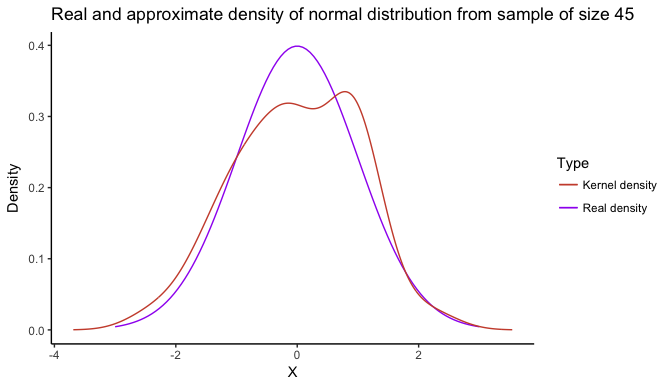There are several kernel types that provide different forms of approximation. For example, consider the following sample:

set.seed(46)
X <- rlnorm(25)

Whose density can be approximated via kernels: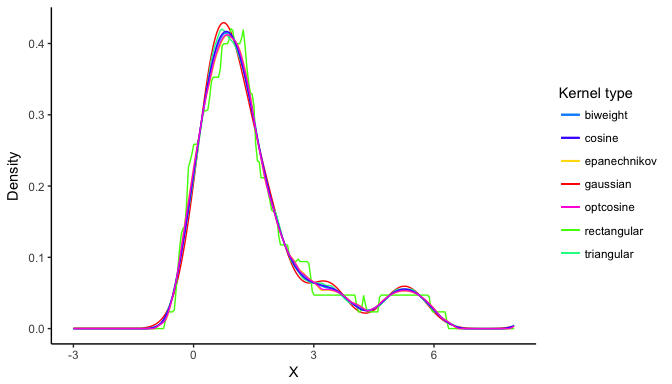Notice that different kernels have different approximations to the sample’s density. Henceforth, if we were to estimate the Potential Impact Fraction, different values would result from different kernels:

X        <- as.data.frame(X)
thetahat <- 1
thetavar <- 0.1
rr       <- function(X, theta){theta*X + 1}
cft      <- function(X){X/2}

#Rectangular kernel
pif.confidence(X, thetahat, rr, cft = cft, method = "kernel", ktype = "gaussian", thetavar = thetavar, nsim = 200)
##           Lower_CI     Point_Estimate           Upper_CI
##        0.266269402        0.323239368        0.436891709
## Estimated_Variance
##        0.002348886
#Gaussian kernel
pif.confidence(X, thetahat, rr, cft = cft, method = "kernel", ktype = "rectangular", thetavar = thetavar, nsim = 200)
##           Lower_CI     Point_Estimate           Upper_CI
##        0.262029974        0.323262140        0.440866975
## Estimated_Variance
##        0.002647825

Additional kernel options include bandwith, adjustment, and number of interpolation points. These options are taken directly from the density function.

pif.confidence(X, thetahat, rr, cft = cft, method = "kernel", ktype = "rectangular", bw = "nrd", adjust = 2, n = 1000, thetavar = thetavar, nsim = 150)
##           Lower_CI     Point_Estimate           Upper_CI
##        0.252420678        0.323258190        0.442930589
## Estimated_Variance
##        0.002480512

#### Approximate

As stated previously, the approximate method should only be used if the only information known to the researcher is sample mean and variance but the sample is not available. The approximate method works with numerical derivatives from the numDeriv package inheriting its options for derivatives. Consider the theoretical function:

rr <- function(X, theta){ theta*X^2/(X + 1) + theta*X + 1}

Assume the following information is available for $$X$$:

Xmean    <- as.data.frame(0.365)
Xvar     <- 0.25
thetahat <- c(0.32, 1/4)

The approximate PAF is given by:

paf(Xmean, thetahat, rr, Xvar = Xvar, method = "approximate")
##  0.1334019

Additional options can be changed to improve the derivation method:

paf(Xmean, thetahat, rr, Xvar = Xvar, method = "approximate", deriv.method = "Richardson", deriv.method.args = list(eps=0.03, d=0.0001, zero.tol=1.e-8, r=4, v=2))
##  0.1334004

### Deciding on the confidence interval

By default, confidence intervals for the empirical and kernel methods are bootstrap; for the approximate method default is loglinear. When calculating confidence intervals for the Population Attributable Fraction additional methods are available: "one2one" and "inverse". #### Force min

The force.min option of "inverse" confidence method forces the Population Attributable Fraction’s interval to be > 0. This option is not recommended as it artificially reduces the uncertainty around estimates.

X <- as.data.frame(rnorm(100))
paf.confidence(X, 0.12, rr = function(X, theta){exp(theta*X)}, thetavar = 0.1, check_exposure = F, confidence_method = "inverse",force.min = FALSE, nsim = 200)
##       Lower_CI Point_Estimate       Upper_CI
##   -0.222421930   -0.002990836    0.177051235
paf.confidence(X, 0.12, rr = function(X, theta){exp(theta*X)}, thetavar = 0.1, check_exposure = F, confidence_method = "inverse", force.min = TRUE, nsim = 200)
##       Lower_CI Point_Estimate       Upper_CI
##   4.726225e-05  -2.990836e-03   1.583408e-01

However, there might be cases for which such a confidence interval makes sense.

### Working with the plots

#### Plot for different values of $$\theta$$

The command pif.plot (paf.plot respectively) allows us to analyze how the PIF (resp. PAF) varies as the values of $$\theta$$ changes:

X        <- as.data.frame(rbeta(100, 1, 3))
rr       <- function(X, theta){theta*X^2 + 1}
cft      <- function(X){X/1.2}
thetalow <- 0
thetaup  <- 5
pif.plot(X = X, thetalow = thetalow, thetaup = thetaup, rr = rr, cft = cft, mpoints = 25, nsim = 15)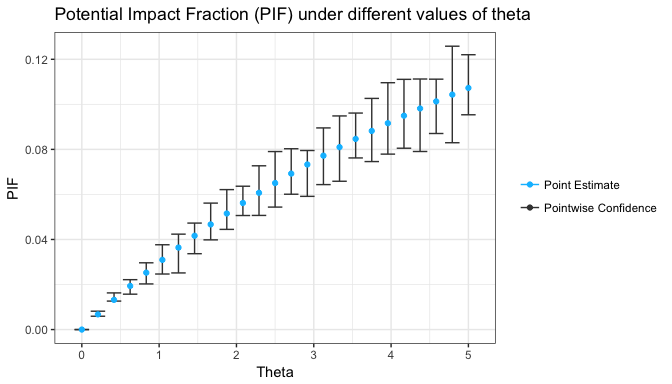Methods can be specified as in pif:

pif.plot(X = X, thetalow = thetalow, thetaup = thetaup, rr = rr, cft = cft, method = "kernel", n = 1000, adjust = 2, ktype = "triangular", confidence_method = "bootstrap", confidence = 99,
mpoints = 25, nsim = 15)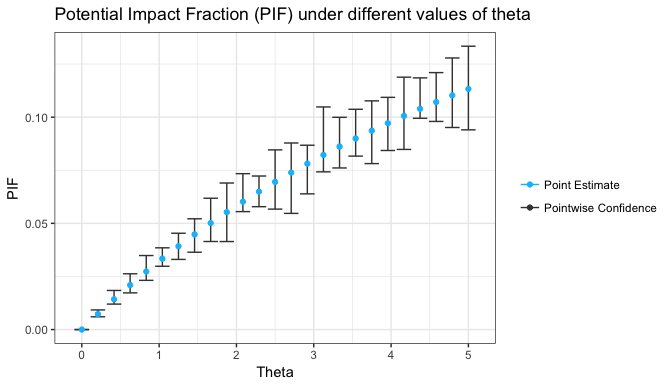Plot options include color and label titles:

pif.plot(X = X, thetalow = thetalow, thetaup = thetaup, rr = rr, cft = cft, colors = rainbow(2), xlab = "Exposure to hideous things.", ylab = "PIF PIF PIF!", title = "This analyisis is the best", mpoints = 25, nsim = 15)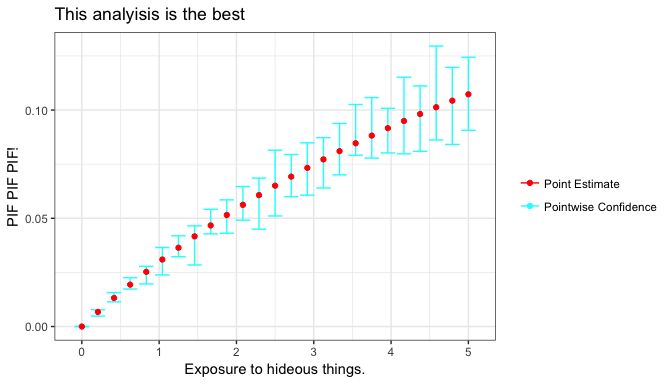pif.plot is a ggplot object and thus one can work with it as one:

#require(ggplot2)
pif.plot(X = X, thetalow = thetalow, thetaup = thetaup, rr = rr, cft = cft, colors = rainbow(2),
mpoints = 25, nsim = 15) + theme_dark()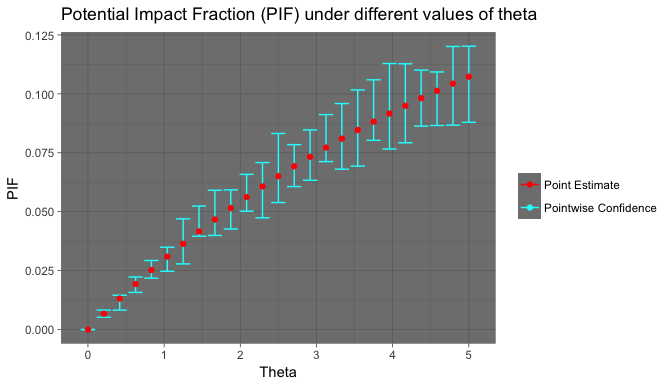#### Sensitivity Analysis

The command pif.sensitivity (paf.sensitivity respectively) allows us to analyze how our estimates for the PIF (resp. PAF) would vary if we excluded some part of the exposure sample, the usage would be the following:

#Get sample
X        <- as.data.frame(sample(c("Exposed","Very exposed","Unexposed"), 540,
replace = TRUE, prob = c(0.25, 0.05, 0.7)))

#Theta values
thetahat <- c(1.2, 7)

#RR defined for each category
rr       <- function(X, theta){
Xnew <- matrix(1, ncol = ncol(X), nrow = nrow(X))
Xnew[which(X[,1] == "Exposed"),1]      <- theta
Xnew[which(X[,1] == "Very exposed"),1] <- theta
return(Xnew)
}

#Counterfactual of stopping the very exposed
cft      <- function(X){
Xcft                                   <- X
Xcft[which(X[,1] == "Very exposed"),] <- "Unexposed"
return(Xcft)
}

#Sensitivity analysis takes some time.
pif.sensitivity(X = X, thetahat = thetahat, rr = rr, cft = cft, mremove = 18, nsim = 10)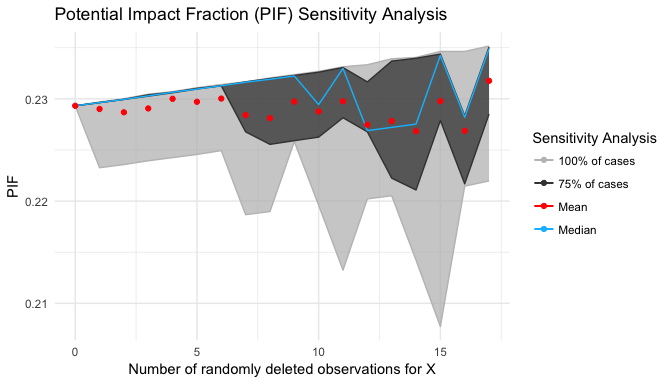The default sensitivity analysis removes mremove elements from the sample X and re-calculates the pif with them nsim times. It is possible to modify those parameters:

pif.sensitivity(X = X, thetahat = thetahat, rr = rr, cft = cft, nsim = 10, mremove = 18)